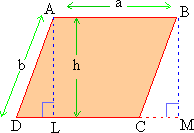Home MonkeyNotes Printable Notes Digital Library Study Guides Message Boards Study Smart Parents Tips College Planning Test Prep Fun Zone Help / FAQ How to Cite New Title Request

 4.4 Parallelogram In a parallelogram the lengths of the opposite sides are equal. Consider the parallelogram ABCD ( figure 4.3).Figure 4.3 seg. AL and seg. BM are perpendiculars on the line containing CD. l (AL) is altitude of the parallelogram. l (AB) = a l (AD) = b l (AL) = h l (BM) = h Consider D ALD and D BMC. Both are right triangles. l (AD) = l (BC) opposite sides of a parallelogram. l (AL) = l (BM) altitudes of a parallelogram. \ D ALD @ D BMC Therefore areas of these two triangles are equal. Consider parallelograms ABCD and ABML. Their areas are equal. Parallelogram ABML is a rectangle. Therefore area of ABML = a ´ h. Thus area of a parallelogram is a product of one base and its corresponding altitude. A = ah The perimeter of the parallelogram = 2 ( a + b ). Index 4.1 Perimeter 4.2 Square 4.3 Rectangle 4.4 Parallelogram 4.5 Triangle 4.6 Trapezoids 4.7 Circles Chapter 5
 Search: All Products Books Popular Music Classical Music Video DVD Toys & Games Electronics Software Tools & Hardware Outdoor Living Kitchen & Housewares Camera & Photo Cell Phones Keywords: